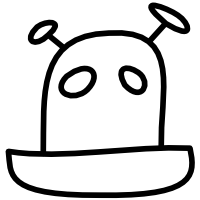# Robot Has No Heart

## Xavier Shay blogs here## Conway's Game of Life in Haskell

Today I came across this excellent game of life implementation in Clojure, and also was learning about monads in Haskell. So I ported the former, using the latter!

The logic translates pretty much the same. Wondering if there is more monads to be had on the `newCell` assignment line (the one with `concatMap` and friends), even at the expense of readability. This is a learning exercise, after all. I went for bonus points by writing a function to render the grid, it didn’t go as well. Would love some feedback on it. Here is a forkable version.

 ```1 2 3 4 5 6 7 8 9 10 11 12 13 14 15 16 17 18 19 20 21 22 23 24 25 26 27 28 29 30 31 32 33 34 35 36 37 38 39 40 41 42 43 44 45 46 47 48 49 50 51 52 53 54 55 56 57 58 59 60 61 62 63 ``` ```import Data.List import Control.Monad type Cell = (Int, Int) type Grid = [Cell] -- Game Logic neighbours :: Cell -> Grid neighbours (x, y) = do dx <- [-1..1] dy <- [-1..1] guard (dx /= 0 || dy /= 0) return (x + dx, y + dy) step :: Grid -> Grid step cells = do (newCell, n) <- frequencies \$ concatMap neighbours cells guard \$ (n == 3) || (n == 2 && newCell `elem` cells) return newCell -- This is the only deviation from the Clojure version, since it is not a -- built-in in Haskell. frequencies :: Ord a => [a] -> [(a, Int)] frequencies xs = do x <- group \$ sort xs return (head x, length x) -- UI -- Feel like I'm missing a concept. Not so happy with this function: -- * Can `eol` be done a better way? I tried nested maps but it was urgh. -- * `marker` seems long for a simple tenary. Same issue as `eol` I guess. formatGrid :: Grid -> String formatGrid grid = do y <- ys x <- xs [marker x y] ++ eol x where marker x y | (x, y) `elem` grid = '*' | otherwise = ' ' eol x | x == maximum xs = ['\n'] | otherwise = [] xs = gridRange fst ys = gridRange snd gridRange f = [min grid .. max grid] where min = minimum . map f max = maximum . map f main = do mapM_ printGrid . take 3 \$ iterate step beacon where beacon = [(0, 0), (1, 0), (0, 1), (3, 3), (2, 3), (3, 2)] printGrid :: Grid -> IO () printGrid grid = do putStrLn \$ formatGrid grid putStrLn "" ```